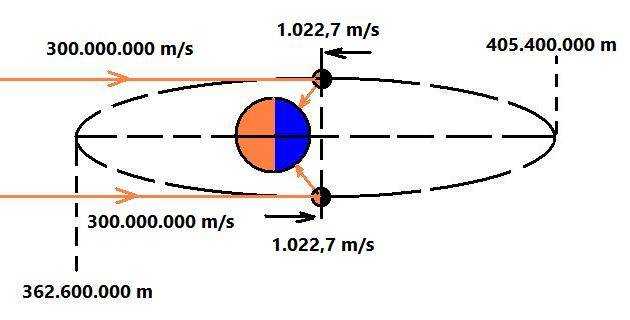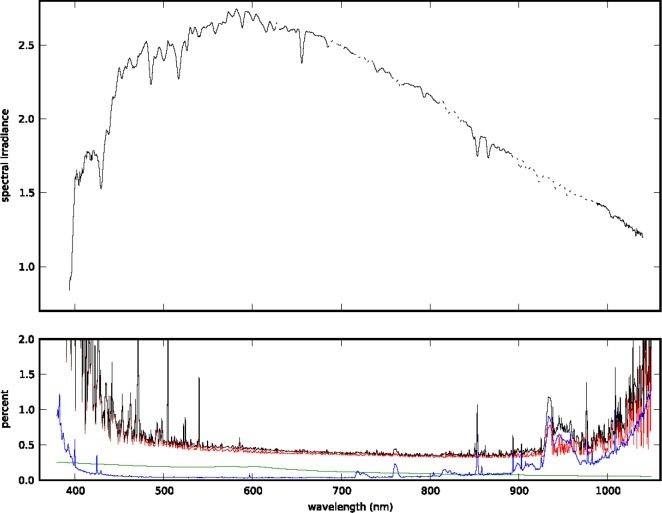# The Colors of The Moon (Doppler Effect)

dom_quixote
Hey guys!

I will pass an illustrated problem, below.- We know that the solar rays that reach the Moon and Earth are practically parallel;

- We know that the lunar orbit with respect to Earth lasts 27.322 days or 2,360,621 seconds;

- We know that the lunar orbit with respect to the Earth is elliptical;

- We know that 27.322 days the distance between the Moon and Earth varies between 362,600 km and 405,400 km.

In order to simplify our calculations, let us consider an average circular orbit:

[405,400,000 m + 362,600,000 m] / 2 = 384,000,000 m

The perimeter of the average lunar orbit is equal to:

2 * pi * 384,000,000 m = 2,412,743,158 m

The speed of the Moon relative to its average orbit is:

2,412,743,158 m / 2,360,621 s = 1,022.7 m/s

Do we have enough technology to detect the Doppler effect of sunlight reflected by the Moon from the Earth's surface?

Gold Member
From your numbers I calculate v/c = 3.4E-6. Balmer spectre of n=3 has wave length of 656 nm would undertake the wave length difference of 2.2 pico meter. I am not good at relevant technologies at all but suppose the effect is too tiny to detect.

•dom_quixote
Keith_McClary
There are some narrow lines:NIST

•dom_quixote and anuttarasammyak